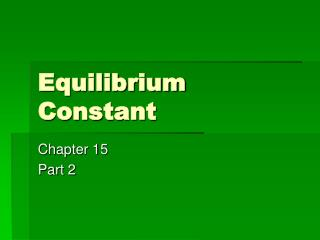DownloadDownload PresentationEquilibrium Constant

# Equilibrium Constant

Télécharger la présentation## Equilibrium Constant

- - - - - - - - - - - - - - - - - - - - - - - - - - - E N D - - - - - - - - - - - - - - - - - - - - - - - - - - -
##### Presentation Transcript

1. Equilibrium Constant Chapter 15 Part 2

2. Review question (conceptual) 2A B • Which of the following must be true of this equilibrium? • (a) K > 1(b) K = 1(c) K < 1(d) K = 0(e) K < 0

3. Applications of equilibrium • Knowing the equilibrium constant allows us to make predictions. • The tendency for the reaction to occur. • If the given concentrations represent equilibrium. • Equilibrium position

4. Assume a Rxn with K=6 • A atoms (red), B atoms (blue), and AB and B2 molecules. • A +B2 AB + B • After the system is at equilibrium, what will the system look like?

5. Write the K expression • 16 = (# AB)(#B) • (#A)(#B2) • Let us pretend: • Initial conditions Final conditions • A = 9 9-5=4 • B2 =12 12-5=7 • AB=0 0+5=5 • B=0 0+5=5

6. Do the new conditions represent equilibrium? • (5) (5) = 0.9 • (4) (7) • Last I looked 0.9 did not equal 16 • Therefore this system is not at equilibrium. • The system needs to move toward products to achieve equilibrium.

7. To reach equilibrium… • A +B2AB + B • For every A that “disappears,” • One AB “appears”. • For every B2 that “disappears”, • One AB and one B appears. • If we call that number X…

8. A +B2AB + B • 16 = (# AB)(#B) = (x)(x) • (#A)(#B2) (9-x)(12-x) • Using Trial & Error • X>5 but less than 9 • Try 8 • (x)(x) = (8)(8) = 64/4 = 16 • (9-x)(12-x) (9-8)(12-8)

9. Extent of a reaction • The tendency of a reaction is indicated by the magnitude of the K • A large K indicates a tendency toward products. • A K much smaller than one indicated a tendency towards the reactants. • However, the magnitude of K and the time to reach equilibrium are not related!!!

10. Reaction Quotient • The Reaction Quotient is used to determine the shift towards or from equilibrium. • It is the same expression, but is not at equilibrium. • It is used for the initial concentrations.

11. Reaction Quotient • When Q = K, the system is at equilibrium. • When Q > K, the system will shift towards the left forming reactants. • When Q < K, the system will shift towards the right forming products.

12. Equilibrium and Gas mixtures

13. Equilibrium and Pressure • Remember [ ] is not just an aqueous situation. • Gas has volume (moles/liter) • Molarity applies to both aqueous and gas mixtures. • PV=nRT • P=(n/V)RT n/V like mol/liter is C • C is concentration

14. Thus P=CRT and if T is constant: • Kc = (CNH3)2/(CN2)(CH2)3 • Kp = (PNH3)2/(PN2)(PH2)3 • K is an equilibrium constant • Kc is about [ ] • Kp is about pressure

15. 2NO(g) + Cl2(g) 2NOCl (g) • At T = 25° C • P NOCl = 1.2 atm • P NO = 5.0 x 10 -2 atm • P Cl2 = 3.0 x 10 -1 atm • Kp = ?? 1.9 x 10 3

16. How do Kp and Kc relate?? • If C= P/RT • Then for N2(g) +3H2(g) 2NH3(g)